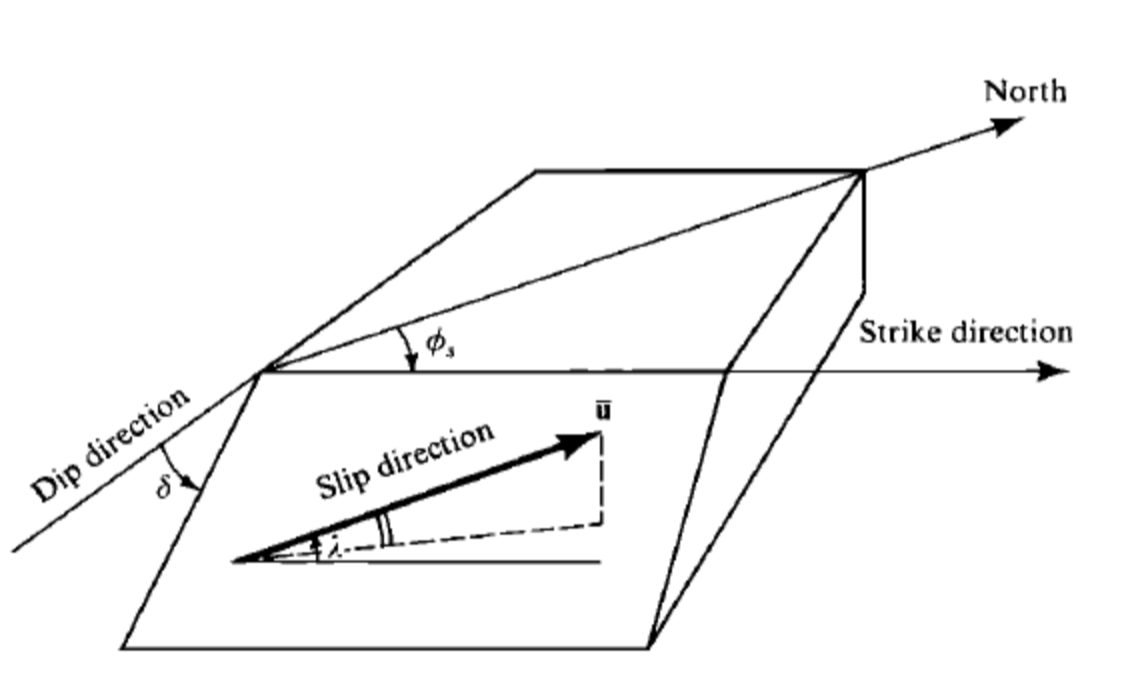# Focal Mechanism¶

## Definition of fault, nodal plane or double couple¶

A double couple source can be describey by three parameters:

1. strike
2. dip
3. rakeDefinition of fault geometry. Figure from P114 of AKi & Richards (1980).

Note that, the coordinate system in Aki & Richards (1980) is NED, North as x direction, East as y direction, Down as z direction.

In NED coordinate system, the fault normal vector can be expressed as:

$\begin{split}\hat{\mathbf{v}} = \left( \begin{array}{c} {-\sin \delta \sin \phi_s} \\ {+\sin \delta \cos \phi_s} \\ {-\cos \delta} \end{array} \right)\end{split}$

the slip vector can be expressed as:

$\begin{split}\hat{\mathrm{u}} = \left( \begin{array}{c} {\cos \lambda \cos \phi_s + \sin \lambda \cos \delta \sin \phi_s} \\ {\cos \lambda \sin \phi_s - \sin \lambda \cos \delta \cos \phi_s} \\ {-\sin \lambda \sin \delta} \end{array} \right)\end{split}$

## How to obtain the auxiliary plane¶

The relationship between the fault and auxiliary planes is:

• the fault normal of fault 1 is the slip vector of fault 2
• the slip vector of fault 1 is the fault normal of fault 2

Thus,

$\begin{split}\left( \begin{array}{c} {\cos \lambda_{1} \cos \phi_{s_{1}} + \sin \lambda_{1} \cos \delta_{1} \sin \phi_{s_{1}}} \\ {\cos \lambda_{1} \sin \phi_{s_{1}} - \sin \lambda_{1} \cos \delta_{1} \cos \phi_{s_{1}}} \\ {- \sin \lambda_{1} \sin \delta_{1}} \end{array} \right) = \left( \begin{array}{c} {-\sin \delta_{2} \sin \phi_{s_{2}}} \\ {\sin \delta_{2} \cos \phi_{s_{2}}} \\ {-\cos \delta_{2}} \end{array} \right)\end{split}$